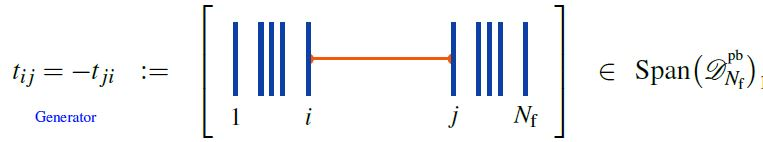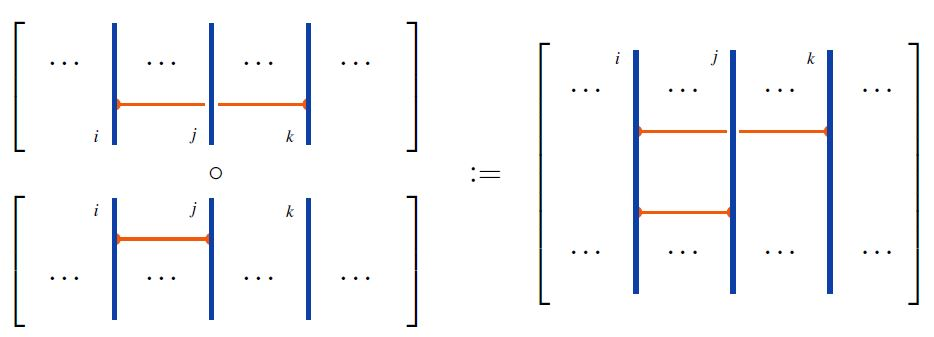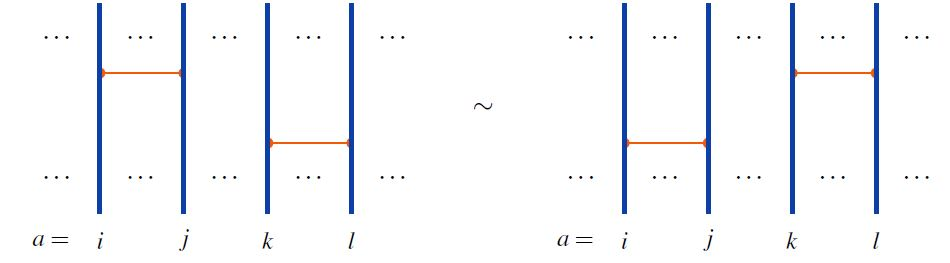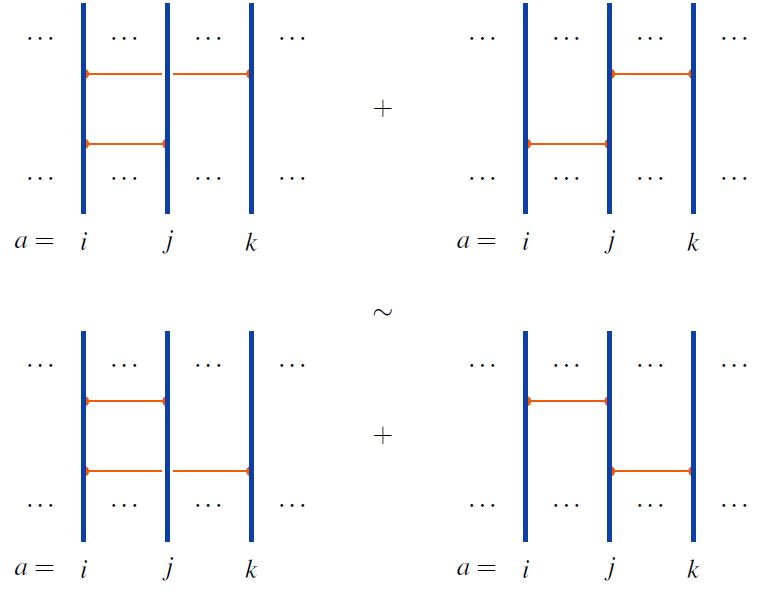# nLab infinitesimal braid relation

Contents

### Context

#### Knot theory

knot theory

Examples/classes:

Types

knot invariants

Related concepts:

category: knot theory

#### Lie theory

Background

Smooth structure

Higher groupoids

Lie theory

∞-Lie groupoids

∞-Lie algebroids

Formal Lie groupoids

Cohomology

Homotopy

Related topics

Examples

$\infty$-Lie groupoids

$\infty$-Lie groups

$\infty$-Lie algebroids

$\infty$-Lie algebras

# Contents

## Definition

###### Definition

(infinitesimal braid Lie algebra)

For $n \in \mathbb{N}$ natural numbers, let $F(\{t_{i j}\}_{i\neq j \in \{1,\cdots, n\}})$ be the free Lie algebra (over a given ground field) on generators $t_{i j}$ in degree with two indices ranging from 1 to $n$ and distinct.

The infinitesimal braid relations in dimension $D = 2$ are the following relations on the underlying vector space of the free Lie algebra on generators $\{t_{i j}\}_{i\neq j \in \{1,\cdots, n\}}$

(1)$\left. \begin{array}{lcll} R0: \;\; & t_{i j} - t_{j i} & = 0 \\ R1: \;\; & [t_{i j}, t_{k l}] & = 0 \\ R2: \;\; & [t_{i j}, t_{i k} + t_{k j}] & = 0 & \mathrlap{\,} \end{array} \right\} \text{for pairwise distinct indices} \;\;$

This defines a quotient Lie algebra often denoted

$\mathcal{L}_n \;\coloneqq\; F(\{t_{i j}\}_{i\neq j \in \{1,\cdots, n\}}) /(R0, R1, R2) \,.$

More generally, there is a version of this in any dimension $D \in \mathbb{N}$ with some extra signs thrown in.

This is originally due to Kohno 87 (1.1.4), it appears also in Kohno 88, Bar-Natan 96, Fact 3, Fadell-Husseini 01, Cohen-Gitler 01, Section 3.

Authors have used different equivalent versions of this presentation, see Cohen-Gitler 02, p. 2 for a clear statement.

## Properties

### Relation to horizontal chord diagrams

If $F(\{t_{i j}\}_{i\neq j \in \{1,\cdots, n\}})/R0$ is identified with the commutator Lie algebra of horizontal chord diagrams on $n$ strands, generated byand forming an associative algebra under concatenation of diagrams along strands, as inthen the remaining infinitesimal braid relations (1) are equivalently the following:

R1 is the the 2T relation:R2 is the 4T relation:graphics from Sati-Schreiber 19c

Hence:

###### Proposition

(universal enveloping algebra of infinitesimal braid Lie algebra is horizontal chord diagrams modulo 2T&4T)

$\Big( \mathcal{A}_n^{pb} \;\coloneqq\; Span \big( \mathcal{D}_n^{pb} \big)/(2T, 4T) , \circ \Big)$

of horizontal chord diagrams on $n$ strands with product given by concatenation of strands (this Def.), modulo the 2T relations and 4T relations (this Def.) is isomorphic to the universal enveloping algebra of the infinitesimal braid Lie algebra (Def. ):

$\big(\mathcal{A}_n^{pb}, \circ\big) \;\simeq\; \mathcal{U}(\mathcal{L}_n(D)) \,.$